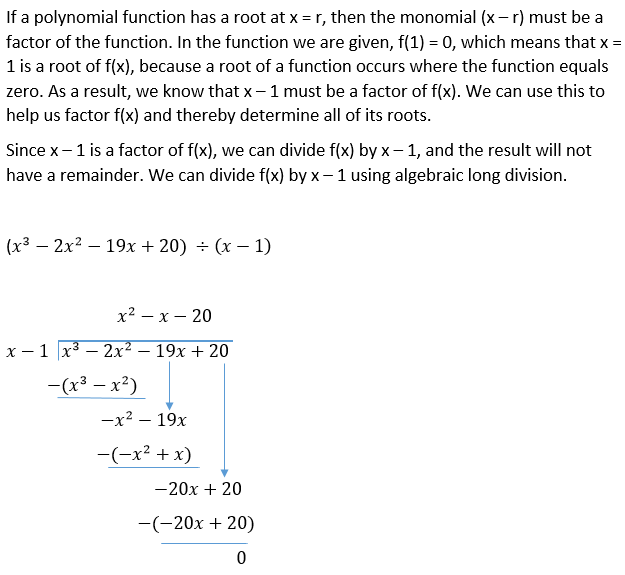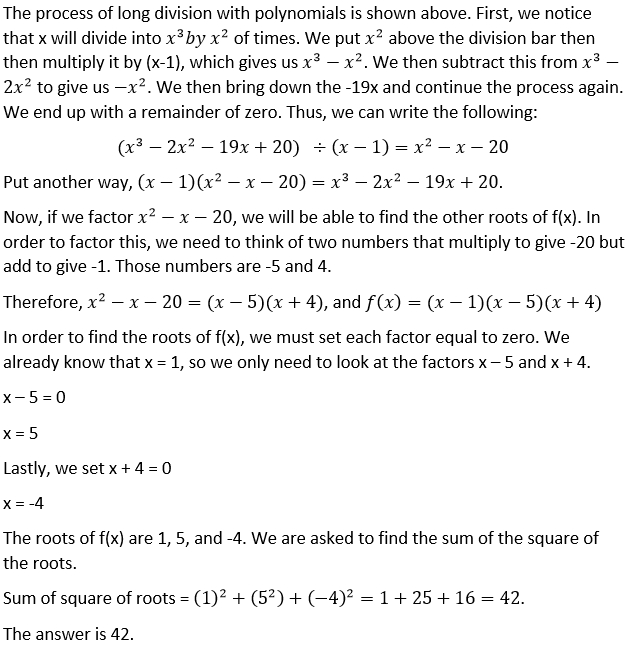## Example Questions

← Previous 1 3 4

### Example Question #1 : How To Find The Degree Of A Polynomial

Find the degree of the polynomial: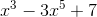Explanation:

To find the degree of a polynomial we must find the largest exponent in the function.

The degree of the polynomialis 5, as the largest exponent ofis 5 in the second term.

### Example Question #2 : How To Find The Degree Of A Polynomial

What is the degree of the polynomial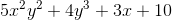?Explanation:

When a polynomial has more than one variable, we need to find the degree by adding the exponents of each variable in each term.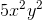has a degree of 4 (since both exponents add up to 4), so the polynomial has a degree of 4 as this term has the highest degree.

### Example Question #3 : How To Find The Degree Of A Polynomial

Find the degree of the following polynomial: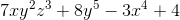Explanation:

When a polynomial has more than one variable, we need to find the degree by adding the exponents of each variable in each term.

Even though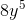has a degree of 5, it is not the highest degree in the polynomial -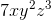has a degree of 6 (with exponents 1, 2, and 3). Therefore, the degree of the polynomial is 6.

### Example Question #1 : How To Find The Degree Of A Polynomial

Solve each problem and decide which is the best of the choices given.

What is the degree of the following polynomial?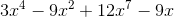Explanation:

The degree is defined as the largest exponent in the polynomial. In this case, it is.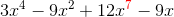### Example Question #5 : How To Find The Degree Of A Polynomial

What is the degree of this polynomial?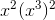Degree 8

Degree 10

Degree 6

Degree 12

Degree 7

Degree 8

Explanation:When an exponent with a power is raised to another power, the value of the power are multiplied.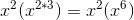When multiplying exponents you add the powers together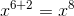The degree of a polynomial is the determined by the highest power. In this problem the highest power is 8.

### Example Question #6 : How To Find The Degree Of A Polynomial

Find the degree of the following polynomial: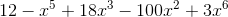Explanation:

The degree of a polynomial is the largest exponent on one of its variables (for a single variable), or the largest sum of exponents on variables in a single term (for multiple variables).

Here, the term with the largest exponent is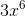, so the degree of the whole polynomial is 6.

### Example Question #21 : Variables

If 3 less than 15 is equal to 2x, then 24/x must be greater than

4

3

6

5

3

Explanation:

Set up an equation for the sentence: 15 – 3 = 2x and solve for x.  X equals 6. If you plug in 6 for x in the expression 24/x, you get 24/6 = 4. 4 is only choice greater than a.

### Example Question #2 : How To Divide Polynomials

Given a♦b = (a+b)/(a-b) and b♦a = (b+a)/(b-a), which of the following statement(s) is(are) true:

I. a♦b = -(b♦a)

II. (a♦b)(b♦a) = (a♦b)2

III. a♦b + b♦a = 0

I and II

I and III

II & III

I, II and III

I only

I and III

Explanation:

Notice that - (a-b) = b-a, so statement I & III are true after substituting the expression. Substitute the expression for statement II gives ((a+b)/(a-b))((a+b)/(b-a))=((a+b)(b+a))/((-1)(a-b)(a-b))=-1 〖(a+b)〗2/〖(a-b)〗2 =-((a+b)/(a-b))2 = -(a♦b)2 ≠ (a♦b)2

### Example Question #1 : How To Divide Polynomials

If a positive integer a is divided by 7, the remainder is 4.  What is the remainder if 3a + 5 is divided by 3?

5

3

6

2

4

2

Explanation:

The best way to solve this problem is to plug in an appropriate value for a.  For example, plug-in 11 for because 11 divided by 7 will give us a remainder of 4.

Then 3a + 5, where = 11, gives us 38.  Then 38 divided by 3 gives a remainder of 2.

The algebra method is as follows:

a divided by 7 gives us some positive integer b, with a remainder of 4.

Thus,

/ 7 = b  4/7

/ 7 = (7b + 4) / 7

a = (7b + 4)

then 3a + 5 = 3 (7+ 4) + 5

(3a+5)/3 = [3(7+ 4) + 5] / 3

= (7+ 4) + 5/3

The first half of this expression (7b + 4) is a positive integer, but the second half of this expression (5/3) gives us a remainder of 2.

### Example Question #4 : How To Divide Polynomials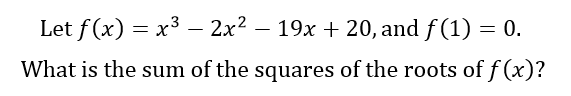45

38

42

36

100